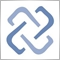Discussion of article "Calculating mathematical expressions (Part 1). Recursive descent parsers"Moderator
245459

New article Calculating mathematical expressions (Part 1). Recursive descent parsers has been published:

The article considers the basic principles of mathematical expression parsing and calculation. We will implement recursive descent parsers operating in the interpreter and fast calculation modes, based on a pre-built syntax tree.

When automating trading tasks, it can be required to provide the flexibility of computational algorithms at their execution stage. For example, when fine tuning programs distributed in a closed (compiled) mode, we can implement the selection of the objective function type from a wide range of possible combinations. In particular, this can be useful when optimizing an Expert Advisor or when quickly evaluating an indicator prototype. In addition to changing parameters in the dialog box, the users will also be able to change the calculation formula. In this case, we need to calculate the arithmetic expression from its textual representation, without changing the MQL program code.

This task can be solved through the use of various parsers allowing formula interpretation on the fly, their "compilation" into a syntax tree, generation of the so-called bytecode (sequence of computational instructions) and its further execution in order to calculate the result. In this article, we will consider several types of parsers and expression calculation methods.

Formulation of the problem

Within this article, an arithmetic expression is a one-line sequence of data items and operators describing the relevant actions. Data items are numbers and named variables. Variable values can be set and edited from the outside, i.e. not in the expression but using special parser attributes. In other words, there is no assignment operator ('=') to store intermediate results. Below is the list of supported operators, shown in calculation priority order:

• !, - , + — unary logical negation, minus and plus
• () — grouping with parentheses
• *, /, % — multiplication, division and division modulo
• +, - — addition and subtraction
• >, <, >=, <= — more-less comparison
• ==, != — equal or not equal comparison
• &&, || — logical AND and OR (please note that priority is the same, so parentheses should be used)
• ?: — ternary conditional operator that allows you to branch calculations according to conditions

We will also allow the usage of MQL standard mathematical functions in expressions, which are 25 in total. One of them is the pow function for exponentiation. For this reason, the list of operators does not have the exponent operator ('^'). In addition, operator '^' only supports an integer power, while the function has no such restrictions. There is one more specific feature that distinguishes '^' from the other considered operators.

Author: Stanislav Korotky